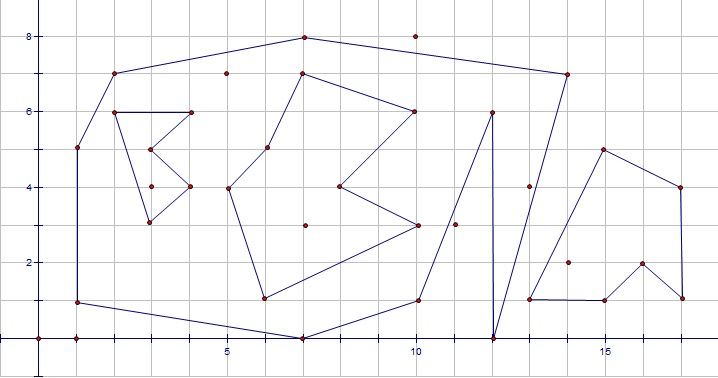Simple Polygons
Tag(s):

## Geometry, Line Sweep Technique, Mathematics, Medium-Hard

Problem
Editorial
Analytics

Given n simple polygons, no two polygons has common points but one polygon can strictly be inside the other. You are asked to answer q queries of two kinds:

1 x y: add a point at position (x, y).

2 u: output the number of points inside or on boundary of u-th polygon.

Input Format

The first line of input contains a single integer T, denoting the number of test cases.

Each test case is described as follow: a single line contains an integer n, denoting the number of polygons, each polygon begins with an integer k, denoting the number of vertices, each of k following lines contains two space-seperated integers x y, denoting coordinates of its vertices. The next line contains an integer q, denoting the number of queries. Each queries is described as above.

Output Format

For each query, output a single line contains the answer.

Constraints

• 1 ≤ T ≤ 5
• 1 ≤ n, q ≤ 105
• 1 ≤ sum of vertices of all polygons overall test cases ≤ 106
• 0 ≤ all coordinates ≤ 108
• 1 ≤ u ≤ n

SAMPLE INPUT
1
4
9
1 1
7 0
10 1
12 6
12 0
14 7
7 8
2 7
1 5
5
3 3
4 4
3 5
4 6
2 6
7
6 1
10 3
8 4
10 6
7 7
6 5
5 4
6
13 1
15 1
16 2
17 1
17 4
15 5
20
1 3 4
2 2
1 5 7
2 2
2 1
1 7 3
2 1
2 2
2 3
1 11 3
1 10 8
1 13 4
2 1
2 2
2 3
1 14 2
2 1
2 2
2 3
2 4


SAMPLE OUTPUT
1
1
2
3
1
1
4
1
1
4
1
1
1

ExplanationTime Limit: 4,0 sec(s) for each input file.
Memory Limit: 512 MB
Source Limit: 1024 KB
Marking Scheme: Marks are awarded when all the testcases pass.
Allowed Languages: Bash, C, C++, C++14, Clojure, C#, D, Erlang, F#, Go, Groovy, Haskell, Java, Java 8, JavaScript(Rhino), JavaScript(Node.js), Julia, Kotlin, Lisp, Lisp (SBCL), Lua, Objective-C, OCaml, Octave, Pascal, Perl, PHP, Python, Python 3, R(RScript), Racket, Ruby, Rust, Scala, Swift, Swift-4.1, TypeScript, Visual Basic

## CODE EDITOR

Initializing Code Editor...

## This Problem was Asked inChallenge Name

March Circuits '18

OTHER PROBLEMS OF THIS CHALLENGE
• Algorithms > Graphs
• Basic Programming > Implementation
• Data Structures > Advanced Data Structures
• Algorithms > Dynamic Programming
• Algorithms > Dynamic Programming
• Math > Number Theory
Уведомления

?